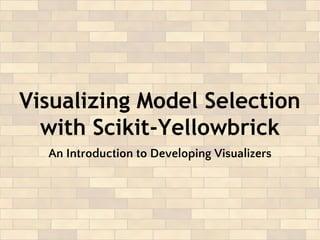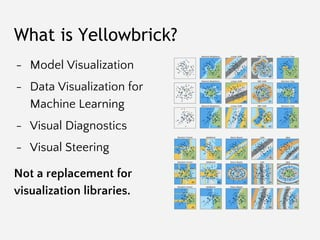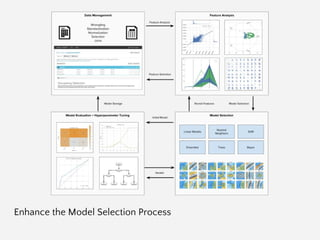Successfully reported this slideshow.

# Visualizing Model Selection with Scikit-Yellowbrick: An Introduction to Developing Visualizers×

1 of 60 Ad
1 of 60 Ad

# Visualizing Model Selection with Scikit-Yellowbrick: An Introduction to Developing Visualizers

This is an overview of the goals and roadmap for the Yellowbrick model visualization library (www.scikit-yb.org). If you're interested in contributing to Yellowbrick or writing visualizers, this is a good place to get started.

In the presentation we discuss the expected workflow of data scientists interacting with the model selection triple and Scikit-Learn. We describe the Yellowbrick API and it's relationship to the Scikit-Learn API. We introduce our primary object: the Visualizer, an estimator that learns from data and displays it visually. Finally we describe the requirements for developing for Yellowbrick, the tools and utilities in place and how to get started.

Yellowbrick is a suite of visual diagnostic tools called "Visualizers" that extend the Scikit-Learn API to allow human steering of the model selection process. In a nutshell, Yellowbrick combines Scikit-Learn with Matplotlib in the best tradition of the Scikit-Learn documentation, but to produce visualizations for your models!

This presentation was given during the opening session of the 2017 Spring DDL Research Labs.

This is an overview of the goals and roadmap for the Yellowbrick model visualization library (www.scikit-yb.org). If you're interested in contributing to Yellowbrick or writing visualizers, this is a good place to get started.

In the presentation we discuss the expected workflow of data scientists interacting with the model selection triple and Scikit-Learn. We describe the Yellowbrick API and it's relationship to the Scikit-Learn API. We introduce our primary object: the Visualizer, an estimator that learns from data and displays it visually. Finally we describe the requirements for developing for Yellowbrick, the tools and utilities in place and how to get started.

Yellowbrick is a suite of visual diagnostic tools called "Visualizers" that extend the Scikit-Learn API to allow human steering of the model selection process. In a nutshell, Yellowbrick combines Scikit-Learn with Matplotlib in the best tradition of the Scikit-Learn documentation, but to produce visualizations for your models!

This presentation was given during the opening session of the 2017 Spring DDL Research Labs.

### Visualizing Model Selection with Scikit-Yellowbrick: An Introduction to Developing Visualizers

1. 1. Visualizing Model Selection with Scikit-Yellowbrick An Introduction to Developing Visualizers
2. 2. What is Yellowbrick? - Model Visualization - Data Visualization for Machine Learning - Visual Diagnostics - Visual Steering Not a replacement for visualization libraries.
3. 3. Enhance the Model Selection Process
4. 4. The Model Selection Process
5. 5. The Model Selection Triple Arun Kumar http://bit.ly/2abVNrI Feature Analysis Algorithm Selection Hyperparameter Tuning
6. 6. The Model Selection Triple - Define a bounded, high dimensional feature space that can be effectively modeled. - Transform and manipulate the space to make modeling easier. - Extract a feature representation of each instance in the space. Feature Analysis
7. 7. Algorithm Selection The Model Selection Triple - Select a model family that best/correctly defines the relationship between the variables of interest. - Define a model form that specifies exactly how features interact to make a prediction. - Train a fitted model by optimizing internal parameters to the data.
8. 8. Hyperparameter Tuning The Model Selection Triple - Evaluate how the model form is interacting with the feature space. - Identify hyperparameters (i.e. parameters that affect training or the prior, not prediction) - Tune the fitting and prediction process by modifying these params.
9. 9. Automatic Model Selection Criteria from sklearn.cross_validation import KFold kfolds = KFold(n=len(X), n_folds=12) scores = [ model.fit( X[train], y[train] ).score( X[test], y[test] ) for train, test in kfolds ] F1 R2
10. 10. Try Them All! from sklearn.svm import SVC from sklearn.neighbors import KNeighborsClassifier from sklearn.ensemble import RandomForestClassifier from sklearn.ensemble import AdaBoostClassifier from sklearn.naive_bayes import GaussianNB from sklearn import cross_validation as cv classifiers = [ KNeighborsClassifier(5), SVC(kernel="linear", C=0.025), RandomForestClassifier(max_depth=5), AdaBoostClassifier(), GaussianNB(), ] kfold = cv.KFold(len(X), n_folds=12) max([ cv.cross_val_score(model, X, y, cv=kfold).mean for model in classifiers ])
11. 11. Search Hyperparameter Space from sklearn.feature_extraction.text import * from sklearn.linear_model import SGDClassifier from sklearn.grid_search import GridSearchCV from sklearn.pipeline import Pipeline pipeline = Pipeline([ ('vect', CountVectorizer()), ('tfidf', TfidfTransformer()), ('model', SGDClassifier()), ]) parameters = { 'vect__max_df': (0.5, 0.75, 1.0), 'vect__max_features': (None, 5000, 10000), 'tfidf__use_idf': (True, False), 'tfidf__norm': ('l1', 'l2'), 'model__alpha': (0.00001, 0.000001), 'model__penalty': ('l2', 'elasticnet'), } search = GridSearchCV(pipeline, parameters) search.fit(X, y)
12. 12. Automatic Model Selection: Search? Search is difficult particularly in high dimensional space. Even with techniques like genetic algorithms or particle swarm optimization, there is no guarantee of a solution. As the search space gets larger, the amount of time increases exponentially.
13. 13. Visual Steering Improves Model Selection to Reach Better Models, Faster
14. 14. Visual Steering - Interventions or guidance by human pattern recognition. - Humans engage the modeling process through visualization. - Overview first, zoom and filter, details on demand.
15. 15. We will show that: - Visual steering leads to improved models (better F1, R2 scores) - Time-to-model is faster. - Modeling is more interpretable. - Formal user testing and possible research paper. Proof: User Testing
16. 16. Yellowbrick Extends the Scikit-Learn API
17. 17. The trick: combine functional/procedural matplotlib + object-oriented Scikit-Learn. Yellowbrick
18. 18. Estimators The main API implemented by Scikit-Learn is that of the estimator. An estimator is any object that learns from data; it may be a classification, regression or clustering algorithm, or a transformer that extracts/filters useful features from raw data. class Estimator(object): def fit(self, X, y=None): """ Fits estimator to data. """ # set state of self return self def predict(self, X): """ Predict response of X """ # compute predictions pred return pred
19. 19. Transformers Transformers are special cases of Estimators -- instead of making predictions, they transform the input dataset X to a new dataset X’. Understanding X and y in Scikit-Learn is essential to being able to construct visualizers. class Transformer(Estimator): def transform(self, X): """ Transforms the input data. """ # transform X to X_prime return X_prime
20. 20. Visualizers A visualizer is an estimator that produces visualizations based on data rather than new datasets or predictions. Visualizers are intended to work in concert with Transformers and Estimators to allow human insight into the modeling process. class Visualizer(Estimator): def draw(self): """ Draw the data """ self.ax.plot() def finalize(self): """ Complete the figure """ self.ax.set_title() def poof(self): """ Show the figure """ plt.show()
21. 21. The purpose of the pipeline is to assemble several steps that can be cross-validated and operationalized together. Sequentially applies a list of transforms and a final estimator. Intermediate steps of the pipeline must be ‘transforms’, that is, they must implement fit() and transform() methods. The final estimator only needs to implement fit(). Pipelines class Pipeline(Transformer): @property def named_steps(self): """ Sequence of estimators """ return self.steps @property def _final_estimator(self): """ Terminating estimator """ return self.steps[-1]
22. 22. Scikit-Learn Pipelines: fit() and predict()
23. 23. Yellowbrick Visual Transformers fit() draw() predict() fit() predict() score() draw()
24. 24. Model Selection Pipelines
25. 25. Primary YB Requirements
26. 26. Requirements 1. Fits into the sklearn API and workflow 2. Implements matplotlib calls efficiently 3. Low overhead if poof() is not called 4. Just flexible enough for users to adapt to their data 5. Easy to add new visualizers 6. Looks as good as Seaborn
27. 27. Primary Requirement: Implement Visual Steering
28. 28. Dependencies Like all libraries, we want to do our best to minimize the number of dependencies: - Scikit-Learn - Matplotlib - Numpy … c’est tout!
29. 29. The Visualizer
30. 30. Current Package Hierarchy: make uml
31. 31. Current Class Hierarchy: make uml
32. 32. Current Class Hierarchy: make uml
33. 33. Current Class Hierarchy: make uml
34. 34. Visualizer Interface Visualizers must hook into the Scikit-Learn API; data is received from the user via: - fit(X, y=None, **kwargs) - transform(X, **kwargs) - predict(X, **kwargs) - score(X, y, **kwargs) These methods then call the internal draw() method. Draw could be called multiple times for different reasons. Users call for visualizations via the poof() method which will: - finalize() - savefig() or show()
35. 35. Visualizer Interface # Instantiate the visualizer visualizer = ParallelCoordinates(classes=classes, features=features) # Fit the data to the visualizer visualizer.fit(X, y) # Transform the data visualizer.transform(X) # Draw/show/poof the data visualizer.poof()
36. 36. Axes Management Multiple visualizers may be simultaneously drawing. Visualizers must only work on a local axes object that can be specified by the user, or created on demand. E.g. no plt.method() calls, use the corresponding ax.set_method() call.
37. 37. A simple example - Create a bar chart comparing the frequency of classes in the target vector. - Where to hook into Scikit-Learn? - What does draw() do? - What does finalize() do?
38. 38. Feature Visualizers FeatureVisualizers describe the data space -- usually a high dimensional data visualization problem! Come before, between, or after transformers. Intersect at fit() or transform()? fit() draw() predict()
39. 39. Some Feature Visualizer Examples
40. 40. Score Visualizers Score visualizers describe the behavior of the model in model space and are used to measure bias vs. variance. Intersect at the score() method. Currently we wrap estimators and pass through to the underlying estimator. fit() predict() score() draw()
41. 41. Score Visualizer Examples
42. 42. Multi-Estimator Visualizers Not implemented yet, but how do we enable visual model selection? Need a method to fit multiple models into a single visualization. Consider hyperparameter tuning examples.
43. 43. Multi-Model visualizations
44. 44. Visual Pipelines
45. 45. Multiple Visualizations How do we engage the pipeline process to add multiple visualizer components? How do we organize visualization with steering? How can we ensure that all visualizers are called appropriately?
46. 46. Interactivity How can we embed interactive visualizations in notebooks? Can we allow the user to tune the model selection process in real time? Do we pause the pipeline process to allow interaction for steering?
47. 47. Features and Utilities
48. 48. Optimizing Visualization Can we use analytics methods to improve the performance of our visualization? E.g. minimize overlap by rearranging features in parallel coordinates and radviz. Select K-Best; Show Regularization, etc.
49. 49. Style Management We should look good doing it! Inspired by Seaborn we have implemented: - set_palette() - set_context() Automatic color code updates: bgrmyck As many palettes and sequences as we can fit!
50. 50. Best Fit Lines Support for automatically drawing best fit lines by fitting a: - Linear polyfit - Quadratic polyfit - Exponential fit - Logarithmic fit
51. 51. Type Detection We’ve had to do a lot of manual work to polish visualizations: - is_estimator() - is_classifier() - is_regressor() - is_dataframe() - is_categorical() - is_sequential() - is_numeric()
52. 52. Exceptions
53. 53. Documentation
54. 54. reStructuredText: cd docs && make html
55. 55. Contributing
56. 56. Git/Branch Management All work happens in develop. Select a card from “ready”, move to “in-progress”. Create a branch called “feature-[feature name]”, work & commit into that branch: \$ git checkout -b feature-myfeature develop Once you are done working (and tested) merge into develop.: \$ git checkout develop \$ git merge --no-ff feature-myfeature \$ git branch -d feature-myfeature \$ git push origin develop Repeat. Once a milestone is completed, it is pushed to master and released.
57. 57. Milestones, Issues, and Labels Each release (identified by semantic versioning; e.g. major and minor releases) is stored in a milestone. Each milestone is a sprint. Issues are added to the milestone, and the release is done with all issues are complete. Issues are labeled for easy categorization.
58. 58. Waffle Kanban
59. 59. Testing (Python 2.7 and 3.5+): make test
60. 60. User Testing and Research